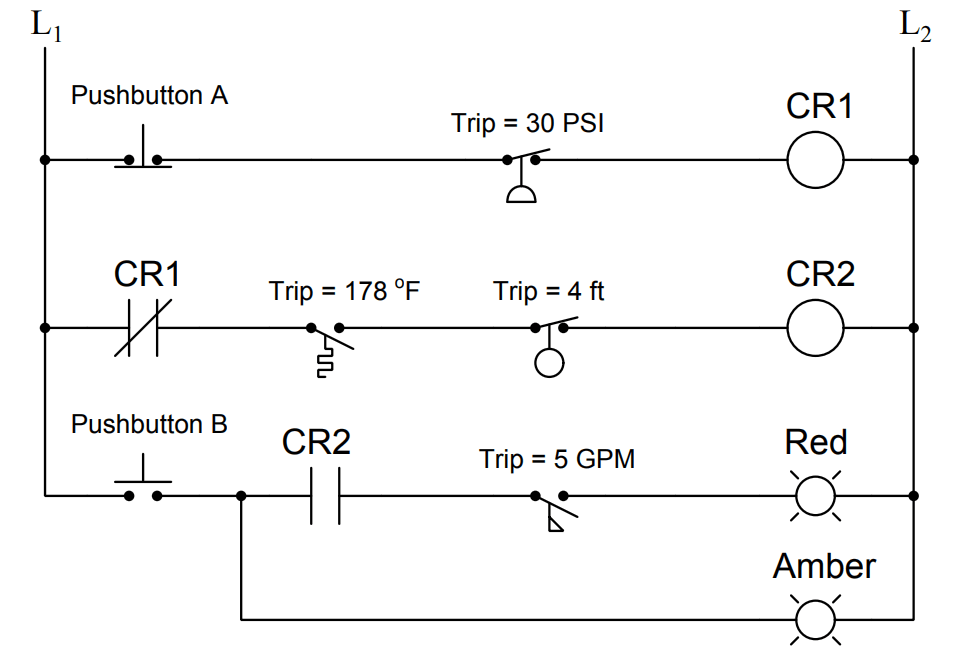# Analyze the Relay Circuit and Find the Status ?

Determine the statuses of all lamps and relay coils in this circuit, given the following process conditions:• Flow = 4 GPM
• Pressure = 24 PSI
• Temperature = 190 Deg F
• Level = 2.5 ft
• Pushbutton A = pressed
• Pushbutton B = pressed

Fully annotated circuit schematic: “X” representing an open contact or de-energized load, and “→” representing a closed contact or energized load.Recall that a process switch will be in its resting (“normal”) condition when the stimulus value is less than the trip threshold, and will be in its actuated condition when the stimulus exceeds the threshold.

Therefore,

1. CR1 coil = de-energized
2. CR2 coil = energized
3. Red lamp = de-energized
4. Amber lamp = energized

Credits : by Tony R. Kuphaldt – under CC BY 1.0